## 5.3.2 Hierarchical Methods

In this section, suppose that two complicated, nonconvex bodies,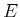and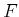, are to be checked for collision. Each body could be part of either the robot or the obstacle region. They are subsets of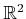or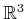, defined using any kind of geometric primitives, such as triangles in. Hierarchical methods generally decompose each body into a tree. Each vertex in the tree represents a bounding region that contains some subset of the body. The bounding region of the root vertex contains the whole body.

There are generally two opposing criteria that guide the selection of the type of bounding region:

1. The region should fit the intended body points as tightly as possible.
2. The intersection test for two regions should be as efficient as possible.
Several popular choices are shown in Figure 5.9 for an L-shaped body.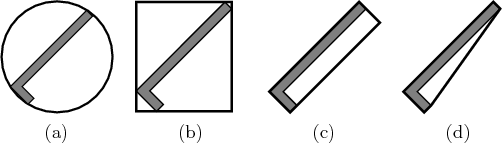The tree is constructed for a body,(or alternatively,) recursively as follows. For each vertex, consider the setof all points inthat are contained in the bounding region. Two child vertices are constructed by defining two smaller bounding regions whose union covers. The split is made so that the portion covered by each child is of similar size. If the geometric model consists of primitives such as triangles, then a split could be made to separate the triangles into two sets of roughly the same number of triangles. A bounding region is then computed for each of the children. Figure 5.10 shows an example of a split for the case of an L-shaped body. Children are generated recursively by making splits until very simple sets are obtained. For example, in the case of triangles in space, a split is made unless the vertex represents a single triangle. In this case, it is easy to test for the intersection of two triangles.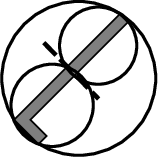Consider the problem of determining whether bodiesandare in collision. Suppose that the trees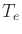and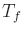have been constructed forand, respectively. If the bounding regions of the root vertices ofanddo not intersect, then it is known thatandare not in collision without performing any additional computation. If the bounding regions do intersect, then the bounding regions of the children ofare compared to the bounding region of. If either of these intersect, then the bounding region ofis replaced with the bounding regions of its children, and the process continues recursively. As long as the bounding regions overlap, lower levels of the trees are traversed, until eventually the leaves are reached. If triangle primitives are used for the geometric models, then at the leaves the algorithm tests the individual triangles for collision, instead of bounding regions. Note that as the trees are traversed, if a bounding region from the vertex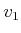ofdoes not intersect the bounding region from a vertex,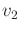, of, then no children ofhave to be compared to children of. Usually, this dramatically reduces the number of comparisons, relative in a naive approach that tests all pairs of triangles for intersection.

It is possible to extend the hierarchical collision detection scheme to also compute distance. The closest pair of points found so far serves as an upper bound that prunes aways some future pairs from consideration. If a pair of bounding regions has a distance greater than the smallest distance computed so far, then their children do not have to be considered . In this case, an additional requirement usually must be imposed. Every bounding region must be a proper subset of its parent bounding region . If distance information is not needed, then this requirement can be dropped.

Steven M LaValle 2012-04-20